Refer to our Texas Go Math Grade 5 Answer Key Pdf to score good marks in the exams. Test yourself by practicing the problems from Texas Go Math Grade 5 Module 2 Assessment Answer Key.

Vocabulary

Choose the best term from the box.

Vocabulary
compatible numbers
partial quotients
place value

Question 1.
You can use _____________ to estimate quotients because they are easy to compute with mentally. (p. 53)
We know that,
You can use “Compatible numbers” to estimate quotients because they are easy to compute with mentally.
Hence, from the above,
We can conclude that
The best term that is suitable for the given statement is: Compatible numbers

Question 2.
To decide where to place the first digit in the quotient you can estimate or use __________. (p. 37)
We know that,
To decide where to place the first digit in the quotient you can estimate or use “Place Value”
Hence, from the above,
We can conclude that
The best term that is suitable for the given statement is: Place Value

Concept and Skills

Estimate. Then find the product. (TEKS 5.3.A, 5.3.B)

Question 3.
Estimate: ____________The given expression is: 576 × 3
Now,
Estimate:
576 × 3 = 580 × 3
= 1,740
Now,
By using the Long Multiplication,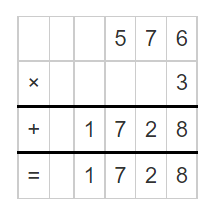Hence, from the above,
We can conclude that
The value of the given expression is: 1,728

Question 4.
Estimate: ____________The given expression is: 219 × 6
Now,
Estimate:
219 × 6 = 220 × 6
= 1,320
Now,
By using the Long Multiplication,Hence, from the above,
We can conclude that
The value of the given expression is: 1,314

Question 5.
Estimate: _____________The given expression is: 72 × 37
Now,
Estimate:
72 × 37 = 70 × 40
= 2,800
Now,
By using the Long Multiplication,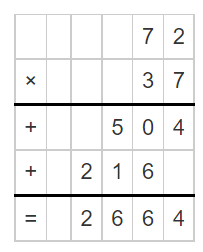Hence, from the above,
We can conclude that
The value of the given expression is: 2,664

Question 6.
Estimate: _____________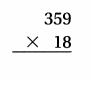The given expression is: 359 × 18
Now,
Estimate:
359 × 18 = 360 × 18
= 6,480
Now,
By using the Long Multiplication,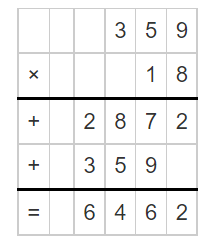Hence, from the above,
We can conclude that
The value of the given expression is: 6,462

Question 7.
Estimate: _____________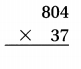The given expression is: 804 × 37
Now,
Estimate:
804 × 37 = 800 × 40
= 32,000
Now,
By using the Long Multiplication,Hence, from the above,
We can conclude that
The value of the given expression is: 29,748

Use compatible numbers to estimate the quotient. (TEKS 5.3. A)

Question 8.
522 ÷ 60
The given division expression is: 522 ÷60
Now,
By using the Long Division,Hence, from the above,
We can conclude that
522 ÷ 60 = 8 R 42

Question 9.
1,285 ÷ 32
The given division expression is: 1,285 ÷ 32
Now,
By using the Long Division,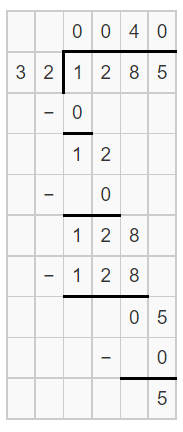Hence, from the above,
We can conclude that
1,285 ÷ 32 = 40 R 5

Question 10.
6,285 ÷ 89
The given division expression is: 6,285 ÷ 89
Now,
By using the Long Division,Hence, from the above,
We can conclude that
6,285 ÷ 89 = 70 R 55

Divide. (TEKS 5.3.C)

Question 11.
156 ÷ 13
The given division expression is: 156 ÷13
Now,
By using the Long Division,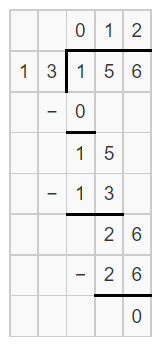Hence, from the above,
We can conclude that
156 ÷ 13 = 12

Question 12.
318 ÷ 53
The given division expression is: 318 ÷ 53
Now,
By using the Long Division,Hence, from the above,
We can conclude that
318 ÷ 53 = 6

Question 13.
1,562 ÷ 34
The given division expression is: 1,562 ÷ 34
Now,
By using the Long Division,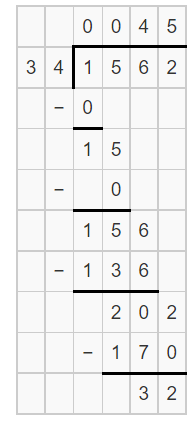Hence, from the above,
We can conclude that
1,562 ÷ 34 = 45 R 32

Question 14.
4,024 ÷ 68
The given division expression is: 4,024 ÷ 68
Now,
By using the Long Division,Hence, from the above,
We can conclude that
4,024 ÷ 68 = 59 R 12

Question 15.
Dylan’s dog weighs 12 times as much as his pet rabbit. The dog and rabbit weigh 104 pounds altogether. How much does Dylan’s dog weigh? (TEKS 5.3.C)
(A) 88 pounds
(B) 96 pounds
(C) 104 pounds
(D) 8 pounds
It is given that
Dylan’s dog weighs 12 times as much as his pet rabbit. The dog and rabbit weigh 104 pounds altogether
Now,
Let the weight of Dylan’s pet rabbit be: x Pounds
So,
The weight of Dylan’s dog is: 12x Pounds
Now,
According to the given information,
12x + x = 104 Pounds
13x = 104 Pounds
x = $$\frac{104}{13}$$
x = 8 Pounds
So,
The weight of Dylan’s dog is: 96 Pounds
Hence, from the above,
We can conclude that
The weight of Dylan’s dog is:Question 16.
Tickets for the basketball game cost $14 each. If the sale of the tickets brought in$2,212, how many tickets were sold? (TEKS 5.3.C)
(A) 172
(B) 158
(C) 150
(D) 168
It is given that
The tickets for the basketball game cost $14 each and the sale of the tickets brought in$2,212
So,
According to the given information,
The number of tickets that were sold = $$\frac{2,212}{14}$$
Now,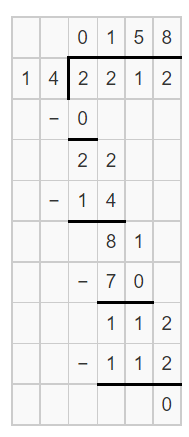Hence, from the above,
We can conclude that
The tickets that were sold are: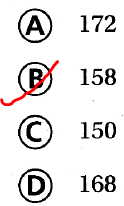Question 17.
Adele earns $194 every two weeks delivering newspapers. She spends$30 of each paycheck and saves the rest. If she is paid 26 times this year, how much will Adele save? (TEKS 5.3.B)
(A) $3,244 (B)$4,264
(C) $772 (D)$5,044
It is given that
Adele earns $194 every two weeks delivering newspapers. She spends$30 of each paycheck and saves the rest. If she is paid 26 times this year
Now,
The amount of money saved by Adele = $194 –$30
= $164 Now, According to the given information, The total amount of money saved by Adele in this year =$164 × 26
Now,Hence, from the above,
We can conclude that
The total amount of money saved by Adele this year is: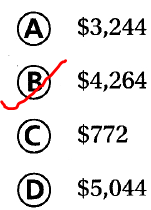Question 18.
A local orange grower processes 2,330 oranges from his grove this year. The oranges are packaged in crates that each holds 96 oranges. All but one crate is full. How many oranges are in this last crate? Record your answer and fill in the bubbles on the grid. Be sure to use the correct place value. (TEKS 5.3.C)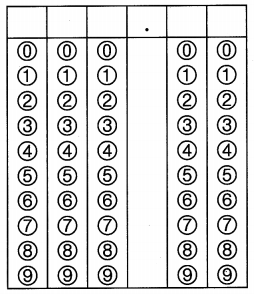It is given that
A local orange grower processes 2,330 oranges from his grove this year. The oranges are packaged in crates that each holds 96 oranges. All but one crate is full
So,
According to the given information,
The number of crates = $$\frac{2,330}{96}$$
Now,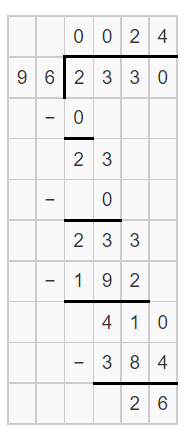So,
The number of oranges that are present in the last crate is: 26 oranges
Hence, from the above,
We can conclude that
The number of oranges that are present in the last crate is:Scroll to Top